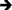## ▼ [Reference]

Tag Link Category [Reference] Technical Substitution No Preferred 6.0 Unchanged Any Reference None LCAPI Lasso 8.5, Lasso 8.0, Lasso 7.0, Lasso 6.0

## ▼ Description

The [Reference] tag allows a reference to data to be stored in a variable rather than a copy of the referenced data. The @ symbol is a synonym for reference.

Every variable in Lasso is a named reference to an object. The referenced object could be a string, integer, array, map, or any other data type. Usually, each object is only referenced by one variable.

It is sometimes desirable to point to the same object using two variables. The same object could be referenced by both a local and page variable. Or, an object could be stored in an array and simultaneously referenced by a page variable.

This is accomplished by storing a reference in a variable. [Variable: 'VariableOne' = (Reference: \$VariableTwo)] stores a reference to VariableTwo in VariableOne. Now, both variables refer to the same object and changes made to one are reflected in the other.

## ▼ Syntax

[Variable: 'VariableReference' = (Reference: \$VariableName)]

[Variable: 'MapReference' = (Reference: \$MapName->(Find: 'Element Name'))]

[Variable: 'ArrayReference' = (Reference: \$ArrayName->(Get: Array Index))]

[Local: 'LocalReference' = (Reference: \$PageVariable)]

[Local: 'ParamReference' = (Reference: Params->(Get: Array Index))]

## ▼ Parameters

Required Parameters
Value The value to be referenced.

## ▼ Examples

To create a reference to an array element:

Use the [Reference] tag. In the following example a reference to the third element of an array is stored in a variable. Changing that variable then changes the third element of the array in-place as demonstrated.

[Variable: 'myArray' = (Array: 'one', 'two', 'three', 'four')]
[Variable: 'myReference' = (Reference: \$myArray->(Get:3))]
[\$myReference = 'new!']
[Output: \$myArray]Array: (one), (two), (new!), (four)